Related Articles

# How to Convert Floats to Strings in Pandas DataFrame?

• Last Updated : 20 Aug, 2020

In this post, we’ll see different ways to Convert Floats to Strings in Pandas Dataframe? Pandas Dataframe provides the freedom to change the data type of column values. We can change them from Integers to Float type, Integer to String, String to Integer, Float to String, etc.

There are three methods to convert Float to String:

Method 1: Using DataFrame.astype().

Syntax :

```DataFrame.astype(dtype, copy=True, errors=’raise’, **kwargs)
```

This is used to cast a pandas object to a specified dtype. This function also provides the capability to convert any suitable existing column to categorical type.

Example 1: Converting one column from float to string.

## Python3

 `# Import pandas library ``import` `pandas as pd `` ` `# initialize list of lists ``data ``=` `[[``'Harvey'``, ``10``, ``45.25``], [``'Carson'``, ``15``, ``54.85``],``        ``[``'juli'``, ``14``, ``87.21``], [``'Ricky'``, ``20``, ``45.23``],``        ``[``'Gregory'``, ``21``, ``77.25``], [``'Jessie'``, ``16``, ``95.21``]] `` ` `# Create the pandas DataFrame ``df ``=` `pd.DataFrame(data, columns ``=` `[``'Name'``, ``'Age'``, ``'Marks'``],``                  ``index ``=` `[``'a'``, ``'b'``, ``'c'``, ``'d'``, ``'e'``, ``'f'``]) `` ` `# lets find out the data type ``# of 'Marks' column``print` `(df.dtypes)`

Output: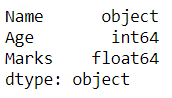Now, we change the data type of column ‘Marks’ from ‘float64’ to ‘object’.

## Python3

 `# Now we will convert it from ``# 'float' to 'String' type. ``df[``'Marks'``] ``=` `df[``'Marks'``].astype(``str``)`` ` `print``()`` ` `# lets find out the data``# type after changing``print``(df.dtypes)`` ` `# print dataframe. ``df `

Output: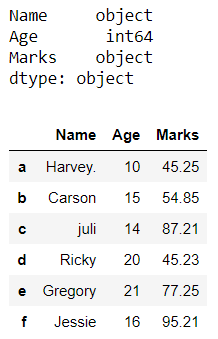Example 2: Converting more than one column from float to string.

## Python3

 `# Import pandas library ``import` `pandas as pd `` ` `# initialize list of lists ``data ``=` `[[``'Harvey.'``, ``10.5``, ``45.25``, ``95.2``], [``'Carson'``, ``15.2``, ``54.85``, ``50.8``], ``        ``[``'juli'``, ``14.9``, ``87.21``, ``60.4``], [``'Ricky'``, ``20.3``, ``45.23``, ``99.5``],``        ``[``'Gregory'``, ``21.1``, ``77.25``, ``90.9``], [``'Jessie'``, ``16.4``, ``95.21``, ``10.85``]] `` ` `# Create the pandas DataFrame ``df ``=` `pd.DataFrame(data, columns ``=` `[``'Name'``, ``'Age'``, ``'Marks'``, ``'Accuracy'``],``                  ``index ``=` `[``'a'``, ``'b'``, ``'c'``, ``'d'``, ``'e'``, ``'f'``]) `` ` `# lets find out the data type ``# of 'Age' and 'Accuracy' columns``print` `(df.dtypes)`

Output: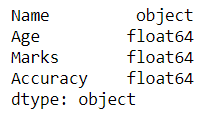Now, we change the data type of columns ‘Accuracy‘ and ‘Age‘ from ‘float64’ to ‘object’.

## Python3

 `# Now Pass a dictionary to ``# astype() function which contains ``# two columns and hence convert them``# from float to string type``df ``=` `df.astype({``"Age"``: ``'str'``, ``"Accuracy"``: ``'str'``})``print``()`` ` `# lets find out the data ``# type after changing``print``(df.dtypes)`` ` `# print dataframe. ``df `

Output: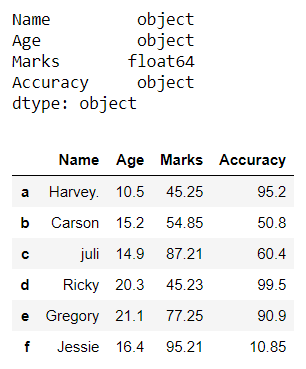Method 2: Using Series.apply().

Syntax :

```DataFrame.apply(func, axis=0, raw=False, result_type=None, args=(), **kwds)
```

This method allows the users to pass a function and apply it on every single value of the Pandas series.

Example: Converting column of a Dataframe from float to string.

## Python3

 `# Import pandas library ``import` `pandas as pd `` ` `# initialize list of lists ``data ``=` `[[``'Harvey.'``, ``10.5``, ``45.25``, ``95.2``], [``'Carson'``, ``15.2``, ``54.85``, ``50.8``],``        ``[``'juli'``, ``14.9``, ``87.21``, ``60.4``], [``'Ricky'``, ``20.3``, ``45.23``, ``99.5``],``        ``[``'Gregory'``, ``21.1``, ``77.25``, ``90.9``], [``'Jessie'``, ``16.4``, ``95.21``, ``10.85``]] `` ` `# Create the pandas DataFrame ``df ``=` `pd.DataFrame(data, columns ``=` `[``'Name'``, ``'Age'``, ``'Percentage'``, ``'Accuracy'``],``                  ``index ``=` `[``'a'``, ``'b'``, ``'c'``, ``'d'``, ``'e'``, ``'f'``]) `` ` `# lets find out the data ``# type of 'Percentage' column``print` `(df.dtypes)`

Output: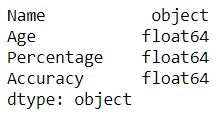Now, we change the data type of column ‘Percentage‘ from ‘float64′ to ”object’.

## Python3

 `# Now we will convert it from ``# 'float' to 'string' type. ``df[``'Percentage'``] ``=` `df[``'Percentage'``].``apply``(``str``) ``print``()`` ` `# lets find out the data``# type after changing``print``(df.dtypes)`` ` `# print dataframe. ``df`

Output: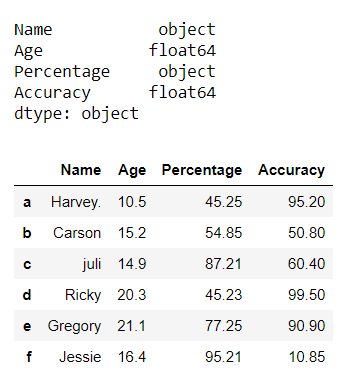Method 3: Using Series.map().

Syntax:

```Series.map(arg, na_action=None)
```

This method is used to map values from two series having one column same.

Example: Converting column of a dataframe from float to string.

## Python3

 `# Import pandas library ``import` `pandas as pd `` ` `# initialize list of lists ``data ``=` `[[``'Harvey.'``, ``10.5``, ``45.25``, ``95.2``], [``'Carson'``, ``15.2``, ``54.85``, ``50.8``], ``        ``[``'juli'``, ``14.9``, ``87.21``, ``60.4``], [``'Ricky'``, ``20.3``, ``45.23``, ``99.5``],``        ``[``'Gregory'``, ``21.1``, ``77.25``, ``90.9``], [``'Jessie'``, ``16.4``, ``95.21``, ``10.85``]] `` ` `# Create the pandas DataFrame ``df ``=` `pd.DataFrame(data, columns ``=` `[``'Name'``, ``'Age'``, ``'Percentage'``, ``'Accuracy'``],``                  ``index ``=` `[``'a'``, ``'b'``, ``'c'``, ``'d'``, ``'e'``, ``'f'``]) `` ` `# lets find out the data``# type of 'Age' column``print` `(df.dtypes)`

Output: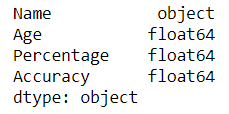Now, we change the data type of column ‘Age‘ from ‘float64′ to ”object’.

## Python3

 `# Now we will convert it from 'float' to 'string' type. ``# using DataFrame.map(str) function``df[``'Age'``] ``=` `df[``'Age'``].``map``(``str``)  ``print``()`` ` `# lets find out the data type after changing``print``(df.dtypes)`` ` `# print dataframe. ``df `

Output: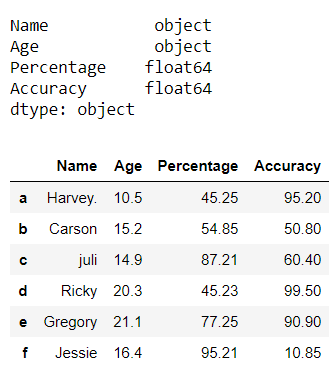Attention geek! Strengthen your foundations with the Python Programming Foundation Course and learn the basics.

To begin with, your interview preparations Enhance your Data Structures concepts with the Python DS Course. And to begin with your Machine Learning Journey, join the Machine Learning – Basic Level Course

My Personal Notes arrow_drop_up